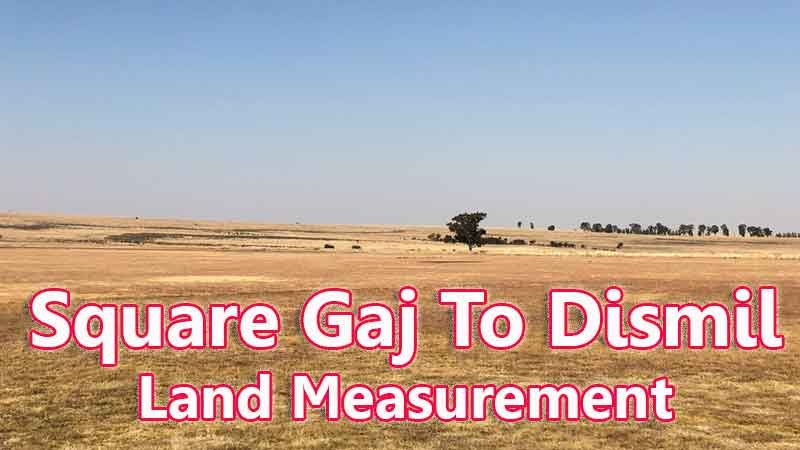# Square Gaj To Dismil Converter

Square Gaj and Dismil come under the land measurement units. Square Gaj is also known as Gaj and is commonly used in the northern part of India like Delhi, Haryana, and Punjab. In Hindi, Gaj is a word that is also used instead of Square Yard. In other words, Gaj is also pronounced as Guz and it was first proposed in the Mughal empire Period in India. Square Gaj is a unit of length and that was a standard measurement unit in Asian Continent like Bangladesh and Nepal.

Input Here

square gaj

Output

dismil

1 square gaj = 0.020661157024793 dismil

## Measurement

The Dismil unit of area is nothing but our familiar Decimal unit because this Dismil is another name for Decimal. This measurement unit is widely used in India and Bangladesh for land measurement stuff. It has also taken place in other countries like East Africa for a measure of land. And this Dismil or Decimal unit has more preference than the standard unit acre because the Dismil unit is mainly used in the state of West Bengal to measure the smaller land layout below the acre. So it was the most used unit in India and Bangladesh for measurement intent. Here drop your value and find the Square Gaj unit to Dismil or Decimal unit by using this calculator.

Square Gaj means - a unit of land measurement. This tool provides great calculation over square gaj to dismil, 1 square gaj to dismil, 1 square gaj in dismil, 1 square gaj equal to and etc. To convert square gaj to dismil, then multiply the unit value by 0.020661157024793. Then, 1 square gaj * 0.020661157024793 = 0.020661157024793 dismil. Hence, 1 square gaj is equal to 0.020661157024793 dismil.

dismil also comes under this land measurement unit and we are comparing values between square gaj traditional unit with other side land measurement unit.

## Formula For square gaj to dismil Conversion(square gaj to dismil formula)

The base formula for this square gaj to dismil converter is

dismil = square gaj * 0.020661157024793

## How many dismil in a square gaj?

Step 1: To Convert 1 square gaj to dismil

Step 2: Applying formula dismil = square gaj * 0.020661157024793, (i.e) multiply the unit value by 0.020661157024793.

Step 3: Then, dismil = 1 * 0.020661157024793 = 0.020661157024793.

Step 4: Hence, 1 square gaj is equal to 0.020661157024793 dismil.Some square gaj to dismil Conversion Chart for your reference:

 1 square gaj = 0.020661157024793 dismil 2 square gaj = 0.04132231404958678 dismil 3 square gaj = 0.06198347107438016 dismil 4 square gaj = 0.08264462809917356 dismil 5 square gaj = 0.10330578512396693 dismil 6 square gaj = 0.12396694214876032 dismil 7 square gaj = 0.1446280991735537 dismil 8 square gaj = 0.1652892561983471 dismil 9 square gaj = 0.1859504132231405 dismil 10 square gaj = 0.20661157024793386 dismil

The square gaj to dismil conversion chart is above listed for your reference. This chart, however, represents the simple math calculation involved in the square gaj to dismil convertion online.

For Example: How much is 1 square gaj to dismil

Solution:

= (square gaj * 0.020661157024793)

= (1 x 0.020661157024793)

= 0.020661157024793 dismil

For Example: How much is 120 square gaj in dismil

Solution:

= (square gaj * 0.020661157024793)

= (120 x 0.020661157024793)

= 2.4793388429752063 dismil

This formula provides an instant answer for all your questions / People also search:

• 1 square gaj to dismil
• 1 square gaj is equal to how many dismil
• Formula to convert square gaj to dismil online
• How to convert square gaj to dismil?
• How much dismil is equal to a one square gaj?
• How many dismil in square gaj?
• 1 square gaj equal to dismil